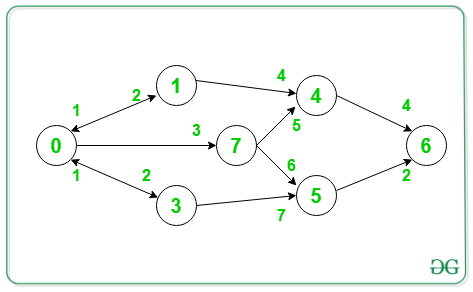# Minimum Cost Path in a directed graph via given set of intermediate nodes

Given a weighted, directed graph G, an array V[] consisting of vertices, the task is to find the Minimum Cost Path passing through all the vertices of the set V, from a given source S to a destination D.

Examples:

Input: V = {7}, S = 0, D = 6Output: 11
Explanation:
Minimum path 0->7->5->6.
Therefore the cost of the path = 3 + 6 + 2 = 11

Input: V = {7, 4}, S = 0, D = 6Output: 12
Explanation:
Minimum path 0->7->4->6.
Therefore the cost of the path = 3 + 5 + 4 = 12

## Recommended: Please try your approach on {IDE} first, before moving on to the solution.

Approach:
To solve the problem, the idea is to use Breadth-First-Search traversal. BFS is generally used to find the Shortest Paths in the graph and the minimum distance of all nodes from Source, intermediate nodes, and the Destination can be calculated by the BFS from these nodes.

Follow the steps below to solve the problem:

• Initialize minSum to INT_MAX.
• Traverse the graph from the source node S using BFS.
• Mark each neighbouring node of the source as the new source and perform BFS from that node.
• Once the destination node D is encountered, then check if all the intermediate nodes are visited or not.
• If all the intermediate nodes are visited, then update the minSum and return minimum value.
• If all the intermediate nodes are not visited, then return minSum.
• Mark the source as unvisited.
• Print the final value of minSum obtained.

Below is the implementation of the above approach:

## C++

 `// C++ Program to implement ` `// the above approach ` `#include ` `using` `namespace` `std; ` ` `  `// Stores minimum-cost of path from source ` `int` `minSum = INT_MAX; ` ` `  `// Function to Perform BFS on graph g ` `// starting from vertex v ` `void` `getMinPathSum(unordered_map<``int``, ` `                                 ``vector > >& graph, ` `                   ``vector<``bool``>& visited, ` `                   ``vector<``int``> necessary, ` `                   ``int` `source, ``int` `dest, ``int` `currSum) ` `{ ` `    ``// If destination is reached ` `    ``if` `(source == dest) { ` `        ``// Set flag to true ` `        ``bool` `flag = ``true``; ` ` `  `        ``// Visit all the intermediate nodes ` `        ``for` `(``int` `i : necessary) { ` ` `  `            ``// If any intermediate node ` `            ``// is not visited ` `            ``if` `(!visited[i]) { ` `                ``flag = ``false``; ` `                ``break``; ` `            ``} ` `        ``} ` ` `  `        ``// If all intermediate ` `        ``// nodes are visited ` `        ``if` `(flag) ` ` `  `            ``// Update the minSum ` `            ``minSum = min(minSum, currSum); ` `        ``return``; ` `    ``} ` `    ``else` `{ ` ` `  `        ``// Mark the current node ` `        ``// visited ` `        ``visited = ``true``; ` ` `  `        ``// Traverse adjacent nodes ` `        ``for` `(``auto` `node : graph) { ` `            ``if` `(!visited[node.first]) { ` ` `  `                ``// Mark the neighbour visited ` `                ``visited[node.first] = ``true``; ` ` `  `                ``// Find minimum cost path ` `                ``// considering the neighbour ` `                ``// as the source ` `                ``getMinPathSum(graph, visited, ` `                              ``necessary, node.first, ` `                              ``dest, currSum + node.second); ` ` `  `                ``// Mark the neighbour unvisited ` `                ``visited[node.first] = ``false``; ` `            ``} ` `        ``} ` ` `  `        ``// Mark the source unvisited ` `        ``visited = ``false``; ` `    ``} ` `} ` ` `  `// Driver Code ` `int` `main() ` `{ ` `    ``// Stores the graph ` `    ``unordered_map<``int``, vector > > ` `        ``graph; ` `    ``graph = { { 1, 2 }, { 2, 3 }, { 3, 2 } }; ` `    ``graph = { { 4, 4 }, { 0, 1 } }; ` `    ``graph = { { 4, 5 }, { 5, 6 } }; ` `    ``graph = { { 5, 7 }, { 0, 1 } }; ` `    ``graph = { { 6, 4 } }; ` `    ``graph = { { 6, 2 } }; ` `    ``graph = { { 7, 11 } }; ` ` `  `    ``// Number of nodes ` `    ``int` `n = 7; ` ` `  `    ``// Source ` `    ``int` `source = 0; ` ` `  `    ``// Destination ` `    ``int` `dest = 6; ` ` `  `    ``// Keeps a check on visited ` `    ``// and unvisited nodes ` `    ``vector<``bool``> visited(n, ``false``); ` ` `  `    ``// Stores intemediate nodes ` `    ``vector<``int``> necessary{ 2, 4 }; ` ` `  `    ``getMinPathSum(graph, visited, necessary, ` `                  ``source, dest, 0); ` ` `  `    ``// If no path is found ` `    ``if` `(minSum == INT_MAX) ` `        ``cout << ``"-1\n"``; ` `    ``else` `        ``cout << minSum << ``'\n'``; ` `    ``return` `0; ` `} `

Output:

```12
```

Time Complexity: O(N+M)
Auxiliary Space: O(N+M)

Attention reader! Don’t stop learning now. Get hold of all the important DSA concepts with the DSA Self Paced Course at a student-friendly price and become industry ready.

My Personal Notes arrow_drop_upCheck out this Author's contributed articles.

If you like GeeksforGeeks and would like to contribute, you can also write an article using contribute.geeksforgeeks.org or mail your article to contribute@geeksforgeeks.org. See your article appearing on the GeeksforGeeks main page and help other Geeks.

Please Improve this article if you find anything incorrect by clicking on the "Improve Article" button below.